## Lab Worksheet 2

We will be working with the msleep data set that is provided with ggplot2. The data set contains information about the sleep habits of 83 mammals. Enter ?msleep on the R console to learn more about the dataset.

head(msleep)
## # A tibble: 6 x 11
##   name  genus vore  order conservation sleep_total sleep_rem sleep_cycle
##   <chr> <chr> <chr> <chr> <chr>              <dbl>     <dbl>       <dbl>
## 1 Chee… Acin… carni Carn… lc                  12.1      NA        NA
## 2 Owl … Aotus omni  Prim… <NA>                17         1.8      NA
## 3 Moun… Aplo… herbi Rode… nt                  14.4       2.4      NA
## 4 Grea… Blar… omni  Sori… lc                  14.9       2.3       0.133
## 5 Cow   Bos   herbi Arti… domesticated         4         0.7       0.667
## 6 Thre… Brad… herbi Pilo… <NA>                14.4       2.2       0.767
## # … with 3 more variables: awake <dbl>, brainwt <dbl>, bodywt <dbl>

Problem 1: Make the following plots: (i) a plot of total time awake vs. body weight, colored by vore (carnivore, herbivore, etc.); (ii) a plot of body weight vs. brain weight, colored by “vore”. When you plot body weight and/or brain weight, consider whether a linear scale or a logarithmic scale seems more appropriate, and explain your reasoning in 1-2 sentences. HINT: Use the functions scale_x_log10() and scale_y_log10() to change the scales.

ggplot(msleep, aes(x = bodywt, y = awake, color = vore)) +
geom_point() +
scale_x_log10(labels = comma)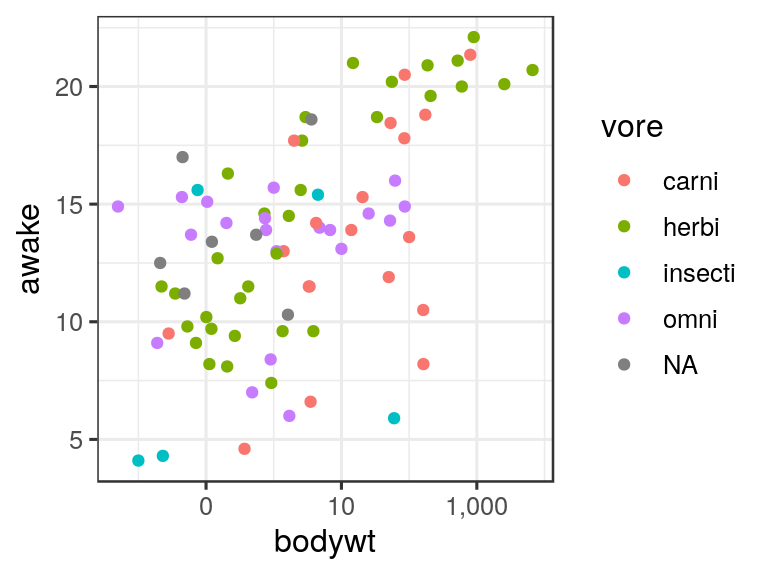ggplot(msleep, aes(x = brainwt, y = bodywt, color = vore)) +
geom_point() +
scale_x_log10() +
scale_y_log10()
## Warning: Removed 27 rows containing missing values (geom_point).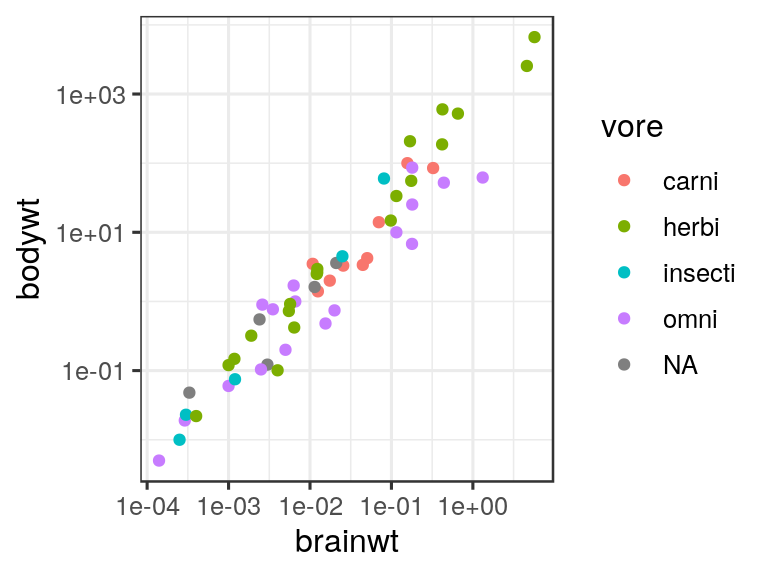Log scales are more appropriate for both body weight and brain weight because there are a few species that have much larger values than most other species. If we were to use a linear scale, these outlying species would require axis ranges that are too wide to resolve any details for the majority of the species.

Problem 2: Plot sleep_total verses bodywt for ONLY carnivores, herbivores, and omnivores. Facet this plot by vore, and then fit a curve to each facet using geom_smooth. In 1-2 sentences, make one observation about total time asleep and body weight.

msleep2 <- msleep %>%
filter(vore == "omni" | vore == "carni" | vore == "herbi")

ggplot(msleep2, aes(x = bodywt, y = sleep_total, color = vore)) +
geom_point() +
geom_smooth() +
facet_wrap(~vore) +
scale_x_log10(label = comma) +
xlab("Body weight (kg)") +
ylab("Total sleep (hours)")
## geom_smooth() using method = 'loess' and formula 'y ~ x'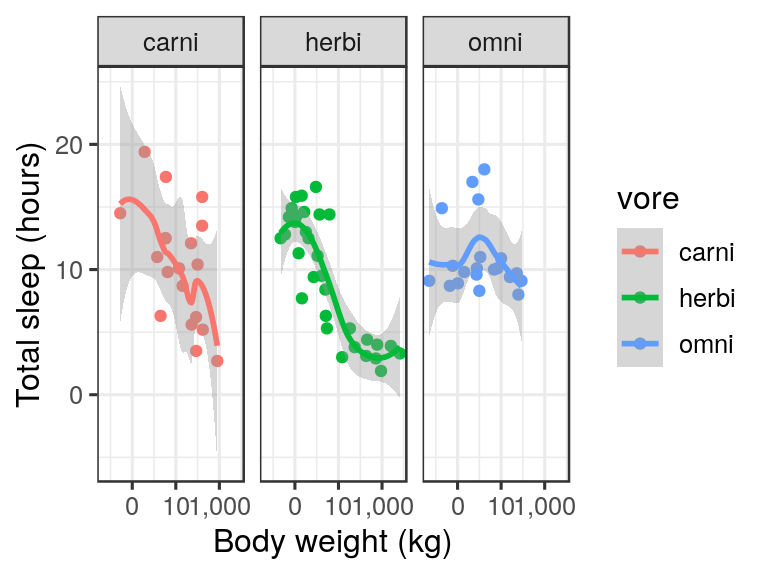Problem 3 (if time): Explain the difference between geom_line() and geom_path(). Make up a simple data set (5-10 data points), plot it twice, once using geom_line() and once using geom_path(), and explain why each plot looks the way it does.

d <- data.frame(x = c(1, 2, 1.5, 1), y = c(1, 1, 2, 1))
ggplot(d, aes(x = x, y = y)) + geom_line()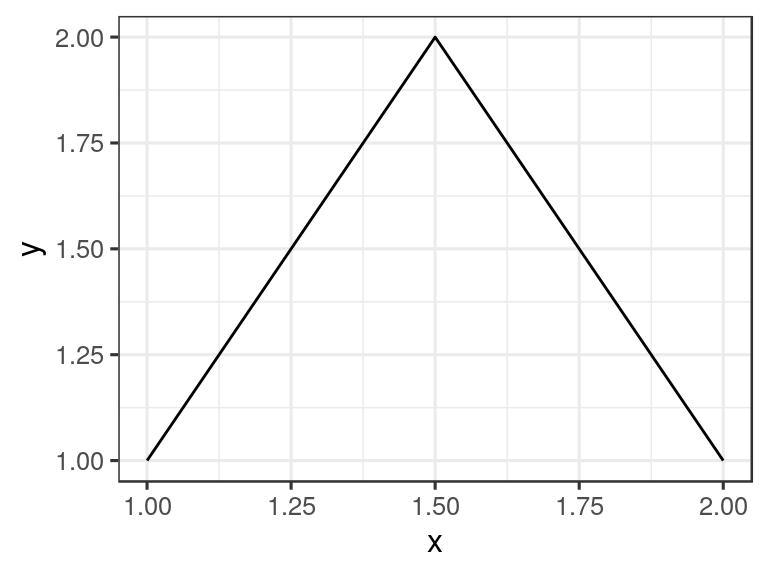ggplot(d, aes(x = x, y = y)) + geom_path()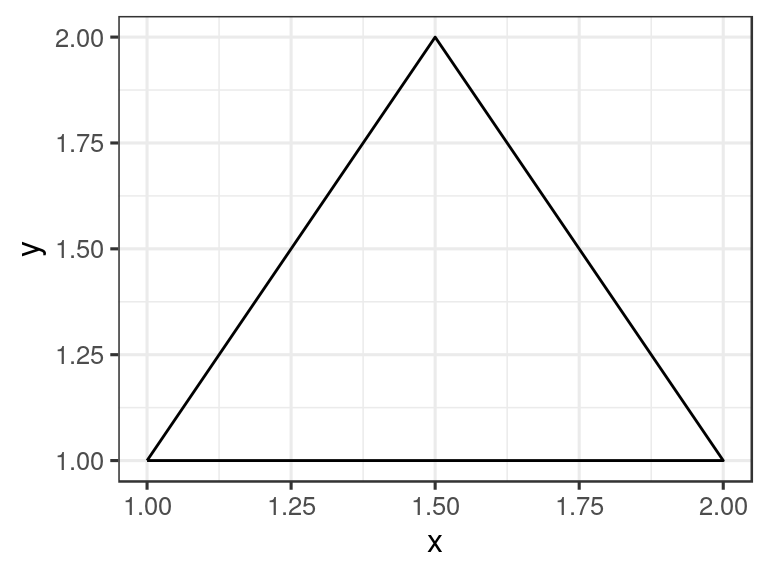geom_line() connects data points in the order from smallest to largest x value. geom_path(), by contrast, connects data points in the order in which they appear in the data frame. The following data set produces an open triangle with geom_line() and a closed triangle with geom_path().